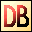DelphiBasics
 ArcCosFunction The Arc Cosine of a number, returned in radians Math unit
 function ArcCos(const Number Extended):Extended;
Description
The ArcCos function is a mathematical function giving the value in radians of the Arc Cosine of Number.

Notes
The System unit supports only the following angular functions:

ArcTan
Sin
Cos

Special floating point values INF, -INF yield +PI/2 and -PI/2 respectively.
Related commands
 ArcSin The Arc Sine of a number, returned in radians ArcTan The Arc Tangent of a number, returned in radians Cos The Cosine of a number Sin The Sine of a number Tan The Tangent of a numberDownload this web site as a Windows program.

 Example code : The ArcCos of 0.5 gives 60 degrees var   float : single; begin   // The ArcCos of 0.5 should give 60 degrees   float := ArcCos(0.5);   float := RadToDeg(float);  // Convert result to degrees   ShowMessage('ArcCos of 0.5 = '+FloatToStr(float)+' degrees'); end; Show full unit code ArcCos of 0.5 = 60 degrees